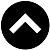-->

Type something and hit enter

# New Structured Warrant Calculator in bursamalaysia.comBy chance I found the above structured warrant calculator in bursamalaysia.com.

But this new calculator does not ask us to input dividend yield.

Is this new method of calculating fair value, which just forget about the dividend yield?

Take an example:

1) Mother price: RM11.00

2) Strike: RM10.00

3) Coversion Ratio: 1

4) Life, n = 0.4 year

5) Interest Rate, i = 3.00%

6) Dividend Yield, D = 2.00%

Assuming I insist to consider also the dividend yield,

So, instead of put in 3.00%, I input ( i - D ) = 3.00% - 2.00% = 1.00% for the Government bill rate.

The fair value I get from the new calculator is RM1.4177 as shown above.

Then I need a scientific calculator to do an adjustment, e^(n*D) = e^(0.4*0.02) = 1.0080321.

The adjusted fair value will be = RM1.4177 / 1.0080321 = RM1.4064.

Let see if I use the old calculator provided by MDEX (Malaysia Derivative Ex-Change),

I get exactly the same RM1.4064.What about my own spreadsheet using 200 steps Binomial Method?

I get the fair value RM1.4072, quite close, can do lah.https://klse.i3investor.com/blogs/gambler/2021-02-13-story-h1541079460-New_Structured_Warrant_Calculator_in_bursamalaysia_com.jsp